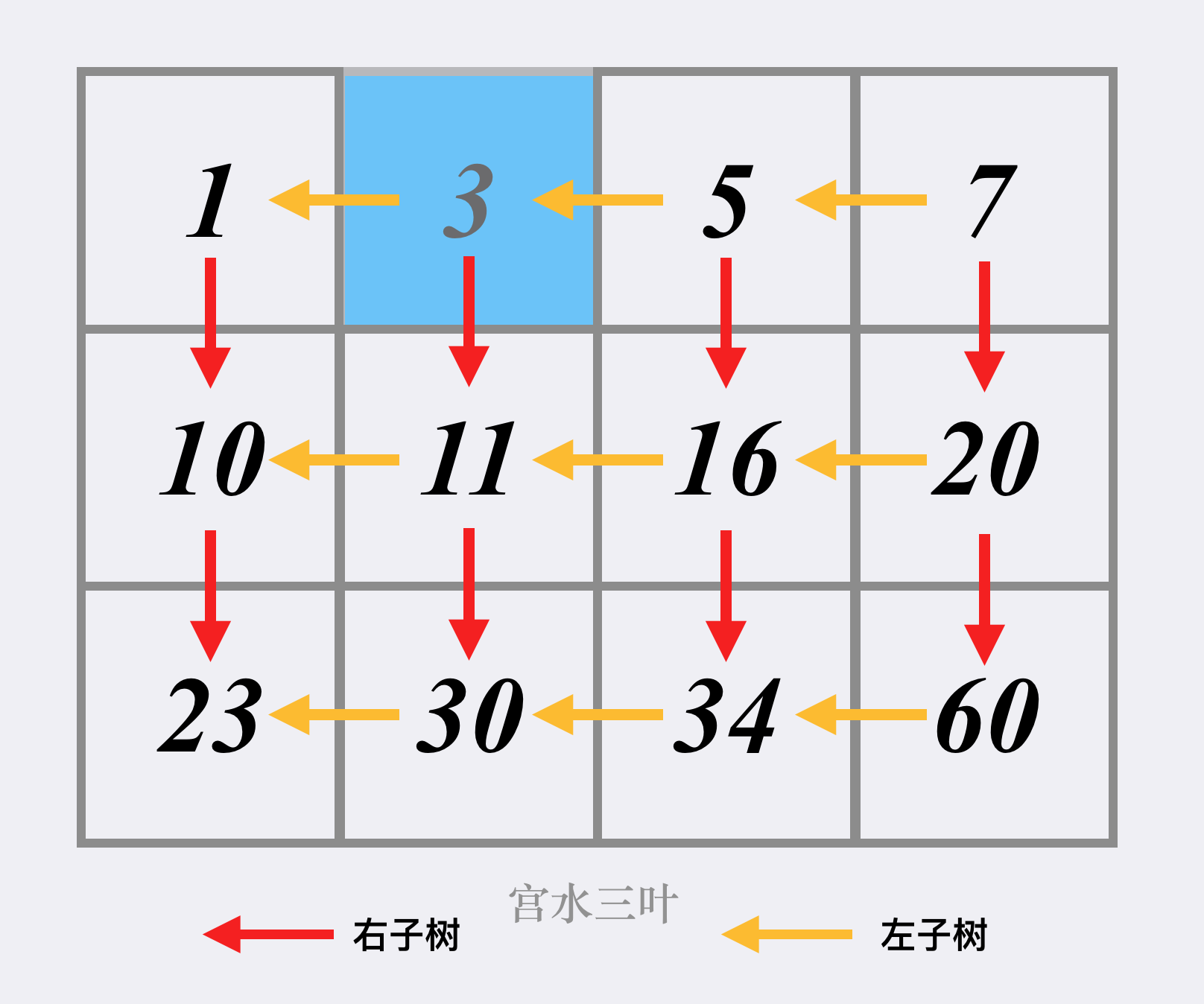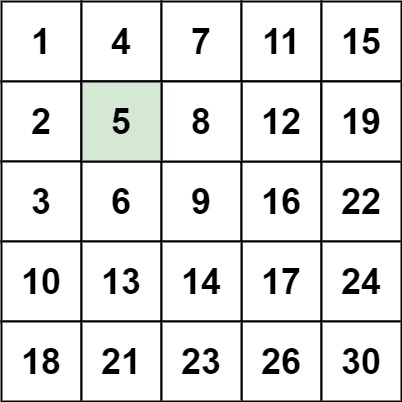# Leetcode 240 - Search a 2d matrix II

Note:

• Use binary search
•• Matrix is just like BST, we start from top right.
• When target === m[i][j], we found the result.
• When target < m[i][j], j-- and we go left.
• When  target < m[i][j], i++ and we go down.

Question:

Write an efficient algorithm that searches for a target value in an m x n integer matrix. The matrix has the following properties:

• Integers in each row are sorted in ascending from left to right.
• Integers in each column are sorted in ascending from top to bottom.

Example:Code: